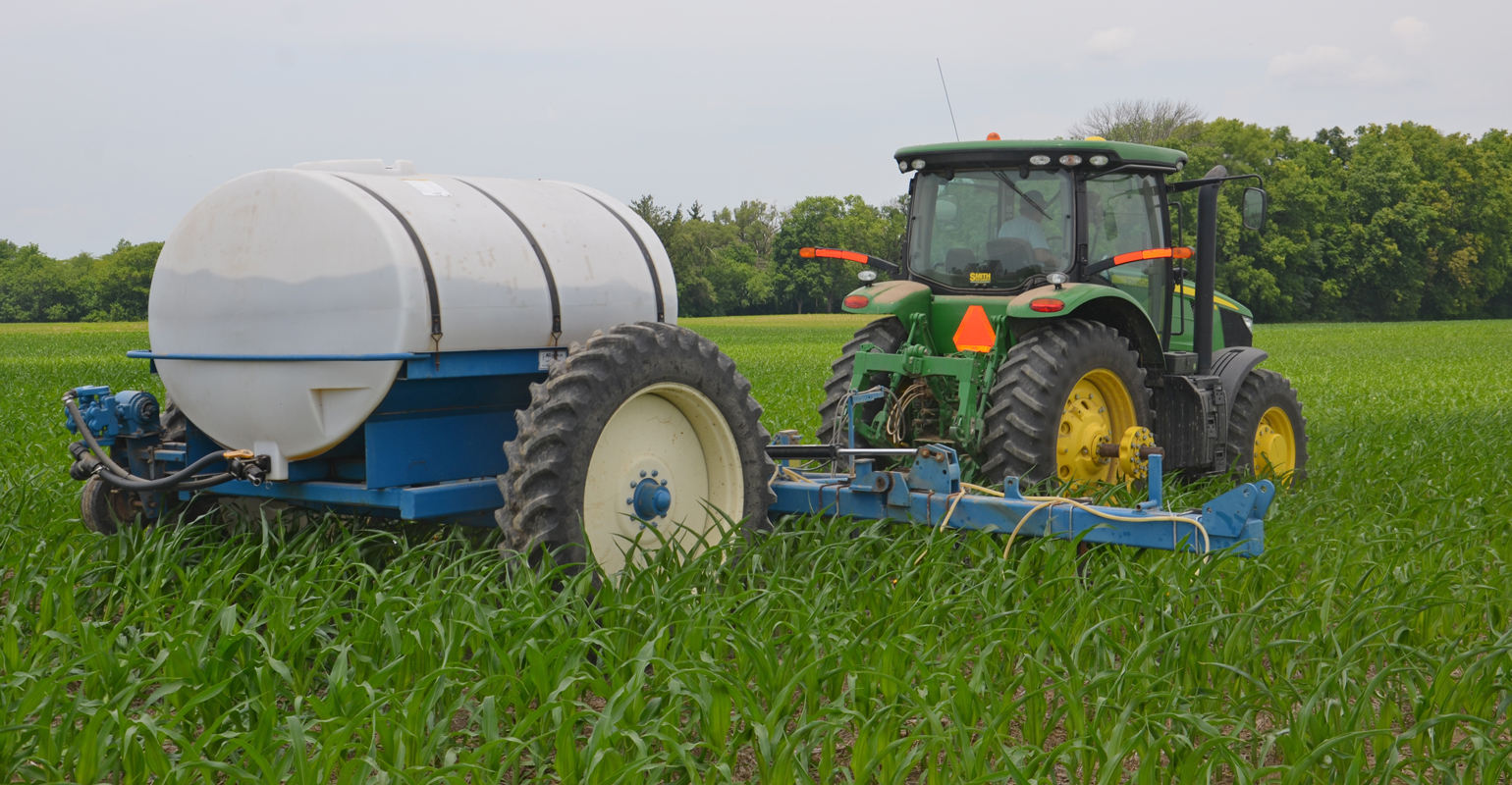# Producing most crop vs. making most profitProducing most crop vs. making most profit

Farm Business: Do you understand the difference between production-maximizing and profit-maximizing input levels?MULTIPLE FACTORS: You may want to adjust final nitrogen rates for corn this season based on nitrogen cost and expected corn price to attempt to maximize profits. Tom J. Bechman

The production-maximizing input level represents the input amount needed for maximum output. For example, for corn, we could compute how much seed or fertilizer would be needed to maximize yield. Agronomists refer to this as the agronomic optimum rate of seed or fertilizer for delivering top yield.

In contrast, the profit-maximizing input level represents the input amount needed to maximize profit. Depending on the input price and output price, the profit-maximizing input level could be substantially different than the production-maximizing input level. It’s important to determine which you’re after when you select input rates.

Before examining a couple of examples, let’s discuss the information needed to compute production- and profit-maximizing input levels. To examine production-maximizing levels, we need output response, such as corn yield, to a specific input, like nitrogen fertilizer. The derivation of profit-maximizing levels requires information pertaining to the output response to a specific input, and input and output prices.

## Seeding rate example

First, we will examine optimum corn seeding rates. Recent work by Bob Nielsen, Dan Quinn and Jim Camberato, Purdue University Extension agronomists, reveals a large difference between the production-maximizing plant population, or agronomic optimum plant population, and the profit-maximizing plant population, or economic optimum plant population.

Based on numerous trials, the production-maximizing plant population was 31,800 plants per acre at harvest. Using a seed cost of \$250 per 80,000 kernels and a corn price of \$6.50 per bushel, the profit-maximizing plant population was 28,334 plants per acre at harvest.

Note that the population determined by years of plant population trials that would net maximum economic return is nearly 3,500 plants per acre fewer than the harvest plant population that would produce highest yield. These are final plant populations. Nielsen usually suggests selecting seeding rates that are about 5% higher.

## Nitrogen rate example

Now, let’s turn our attention to optimum nitrogen rates for corn. Use the N Rate Calculator decision tool and select central Indiana, based on field trials conducted jointly by Purdue Agronomy faculty, agricultural Extension educators and farmers.

Assuming a corn price of \$6.50 per bushel, the optimum economic N rate drops from 211 pounds per acre when N is 50 cents per pound to 191 pounds per acre when N is \$1 per pound. In contrast, the production-maximizing level of nitrogen, the rate needed for highest yield, would be 232 pounds per acre. If you live elsewhere in Indiana, optimum N rates will be either higher or lower.

## Use precision technology

Use profit-maximizing input levels rather than production-maximizing input levels. This is a simple extension of the basic economic concept of comparing benefits to costs.

As price of an output, such as corn, increases, use more of a specific input. Conversely, as cost of an input such as seed or nitrogen increases, use less of that input.

The advent of GPS and other precision agriculture technologies enables you to readily use profit-maximizing levels for different fields or parts of fields. This is particularly important for fields or areas within fields where soil type, fertility level, pH or other factors vary widely.

Langemeier is a Purdue Extension ag economist and associate director of the Purdue Center for Commercial Agriculture.

Subscribe to receive top agriculture news
Be informed daily with these free e-newsletters

You May Also Like